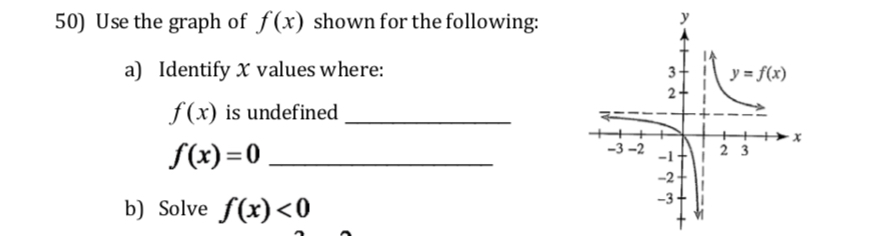# 50) Use the graph of f(x) shown for the following:a)Identify x values where:f(xs undefinedf(x)=0-3-2-2 3b) Solve f(x)

Question
24 viewshelp_outlineImage Transcriptionclose50) Use the graph of f(x) shown for the following: a) Identify x values where: f(xs undefined f(x)=0 -3-2 -2 3 b) Solve f(x) <0 fullscreen
check_circle

Step 1

a) It is clear from graph, if x approaches 1 from left , f(x) approaches -infinity and if x approaches 1 from right , f(x) approaches +infinity. Therefore f(x) is undefined at x=1

also since graph passes through origin, therefore f(x)=0 at x=0

Step 2

Also, for 0<x<1, values...

### Want to see the full answer?

See Solution

#### Want to see this answer and more?

Solutions are written by subject experts who are available 24/7. Questions are typically answered within 1 hour.*

See Solution
*Response times may vary by subject and question.
Tagged in
MathAlgebra

### Other